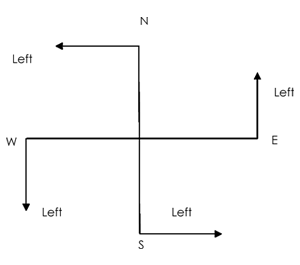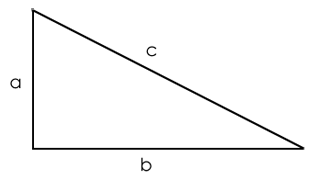# Distances And Directions-Catching Tricks to solve easily(Aptitude)In this article, we are going to discuss how to solve direction sense test questions in reasoning.

##### DIRECTIONS AND DISTANCES

Questions based on this concept are frequently asked in MBA entrance exams like CAT, SNAP, MAT, XAT, etc, Bank PO exams and other aptitude exams.

Directions questions asked in the exam are based on two principles-

• Distance
• Direction

The first step for solving the questions using the concept of ‘directions’ is to understand the direction chart, which has 8 directions. Take a look at the direction chart given below.The first point you need to remember is that each main direction change undergoes a 90° change in direction e.g. from North to West/East it will be 90° change. But the change between North and North-east is only 45°

The next important point that needs to be kept in mind is that directions problems generally quiz you about the minimum distance, distance by air, crow fly distance etc. For the purpose of solving these questions, we assume all these distances to be along straight lines and between specified points. We use the concept of Pythagoras theorem to solve the questions. This concept is discussed in this article.

Left Right Movement

A person facing north, on taking left will face towards west and on taking the right turn towards east.

A person facing west, on taking left will face towards south and on taking right turn towards north.

A person facing east, on taking left will face towards north and on taking the right turn towards south.

A person facing south, on taking left will face towards east and on taking the right turn towards west.Hence, we can sum up the above points as:

• Whenever a person moves to his left side, he will move towards anti- clockwise direction.
• Whenever a person moves to his right side, he will move towards clockwise direction.

<pNote: When a question says moved towards left or right side, we assume that the movement is at an angle of 90 degrees.

Concept of Pythagoras Theorem

We’ll use the concept of Pythagoras theorem to solve the questions on directions.

In a right angled triangle, the square of the hypotenuse is equal to the sum of the squares of the other two sides.c2=a2 + b2

Examples:

Example 1: A man goes 3 kms. East from point A and then takes a right turn from point B to move 4 kms. to point C. What is the minimum distance between point A and point C?

Solution: In order to find the minimum distance between these points, we use a little bit of geometry. We know that the minimum distance between these points will lie along the hypotenuse of the right-angled triangle formed by these points

Now applying Pythagoras theorem, the distance between the starting point A and final point C is 5 kms i.e. the square root of the sum of squares of 3 and 4.An important point to learn from this question could be the fact that you might be asked to specify the direction of the specific point, for example, the question might state: “in which direction is he with respect to the starting point”. The answer would be South-east.
Now, in case the question was: “In which direction is the starting point with respect to C”; the answer would be North-west.

Another question could be: “In which direction is he walking towards point C”; the answer would be South.

Correct! Wrong!

Correct! Wrong!

Correct! Wrong!

Correct! Wrong!

#### Ravi left home and cycled 10 km towards South, then turned right and cycled 5 km and then again turned right and cycled 10 km. After this he turned left and cycled 10 km. How many kilometers will he have to cycle to reach his home straight?

Correct! Wrong!

Distances and directions
Wonderful,you can explore quite good at new places
Very good,Its hard to confuse you while travelling
Good,try to work hard